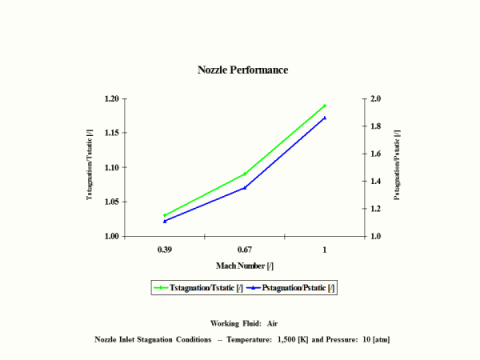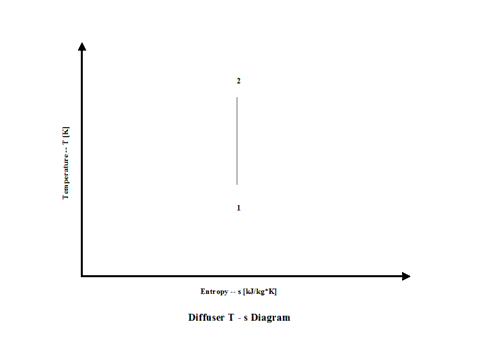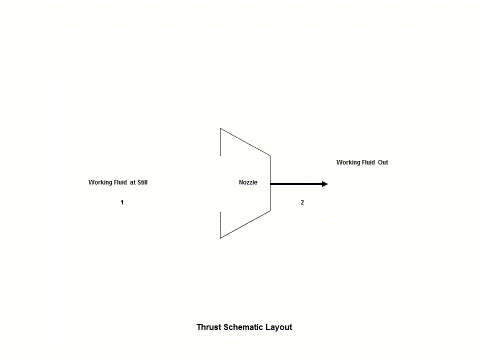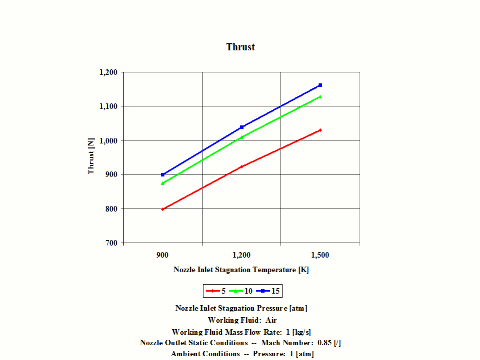top of page

### Compressible Flow Analysis

This section provides free Compressible Flow Analysis.

Compressible Flow Analysis covers the following areas:
Nozzle
Diffuser
Thrust

Nozzle

Introduction

This section provides an isentropic nozzle analysis when the working fluid is air.

Analysis

In the presented nozzle analysis, only air is considered as the working fluid behaving as a perfect gas -- specific heat has a constant value.  Ideal gas state equation is valid -- pv = RT.

Air enters a nozzle at point 1 and it exits the nozzle at point 2.  Isentropic expansion is considered with no entropy change.

Figure 1 contains a nozzle schematic layout.Figure 1 - Nozzle Schematic Layout

Figure 2 presents a nozzle temperature vs entropy diagram.Figure 2 - Nozzle Temperature vs Entropy Diagram

Figure 3 presents nozzle performance -- stagnation over static temperature and pressure ratio values as a function of the Mach Number.  Only subsonic nozzle operation is considered.  It should be noted that air enters the nozzle at the stagnation conditions of 1,500 [K] and 10 [atm] of absolute pressure.Figure 3 - Nozzle Performance

One can notice that nozzle stagnation over static temperature and pressure ratio values increase with an increase in the Mach Number.

Assumptions

Working fluid is air.  There is no friction and heat transfer.  Expansion is isentropic -- there is no entropy change.  Ideal gas state equation is valid -- pv = RT.  Air behaves as a perfect gas -- specific heat has a constant value.

Governing Equations

Tt/T = (1 + M^2(k - 1)/2)
p
t/p = (1 + M^2(k - 1)/2)^
ϰ/(ϰ-1)
T
t/T = (pt/p)^(
ϰ-1)/ϰ
v = (2c
p(Tt - T))1/2
v
s = (
ϰRT)^1/2

M = v/vs

ϰ = cp/cv
c
p - cv = R
pv = RT

Input Data

T1 = 1,500 [K]
p
1 = 10 [atm]
R = 0.2867 [kJ/kg*K]
c
p = 1.004 [kJ/kg*K]

ϰ = 1.4 [/]

M = 0.39, 0.67 and 1 [/]

Results

Nozzle Performance vs Outlet Mach Number
Nozzle Inlet Stagnation Temperature = 1,500 [K] and Pressure = 10 [atm]

Outlet Mach
Number

[/]

0.39

0.67

1.00

Stagnation/Static
Temperature Ratio

[/]

1.03

1.09

1.19

Stagnation/Static
Pressure Ratio

[/]

1.11

1.35

1.86

Conclusions

Nozzle stagnation over static temperature and pressure ratio values increase with an increase in the Mach Number.

References

JANAF Thermochemical Data - Tables, 1970

Diffuser

Introduction

This section provides an isentropic diffuser analysis when the working fluid is air.

Analysis

In the presented diffuser analysis, only air is considered as the working fluid behaving as a perfect gas -- specific heat has a constant value.  Ideal gas state equation is valid -- pv = RT.

Air enters a diffuser at point 1 and it exits the diffuser at point 2.  Working fluid inlet velocity gets reduced to zero resulting in the stagnation temperature and pressure inrease.  Isentropic process is considered with no entropy change.

Figure 1 contains a diffuser schematic layout.Figure 1 - Diffuser Schematic Layout

Figure 2 presents a diffuser temperature vs entropy diagram.Figure 2 - Diffuser Temperature vs Entropy Diagram

Figure 3 presents diffuser performance -- stagnation over static temperature and pressure ratio values as a function of the Mach Number.  Only subsonic diffuser operation is considered.  It should be noted that air enters the diffuser at the static conditions of 298 [K] and 1 [atm] of absolute pressure.Figure 3 - Diffuser Performance

One can notice that diffuser stagnation over static temperature and pressure ratio values increase with an increase in the Mach Number.

Assumptions

Working fluid is air. There is no friction and heat transfer. Isentropic process -- there is no entropy change.  Ideal gas state equation is valid -- pv = RT.  Air behaves as a perfect gas -- specific heat has a constant value.

Governing Equations

Tt/T = (1 + M^2(k - 1)/2)
p
t/p = (1 + M^2(k - 1)/2)^
ϰ/(ϰ-1)
T
t/T = (pt/p)^(
ϰ-1)/ϰ
T
t = T + v^2/(2cp)
v
s = (
ϰRT)^1/2
M = v/v
s

ϰ = cp/cv
cp - cv = R
pv = RT

Input Data

T1 = 298 [K]
p
1 = 1 [atm]
R = 0.2867 [kJ/kg*K]
c
p = 1.004 [kJ/kg*K]

ϰ = 1.4 [/]

M = 0.29, 0.58 and 0.95 [/]

Results

Diffuser Performance vs Inlet Mach Number
Diffuser Inlet Static Temperature = 298 [K] and Presuure = 1 [atm]

Inlet Mach
Number

[/]

0.29

0.58

0.95

Stagnation/Static
Temperature Ratio

[/]

1.017

1.067

1.182

Stagnation/Static
Pressure Ratio

[/]

1.06

1.25

1.80

Conclusions

Diffuser stagnation over static temperature and pressure ratio values increase with an increase in the Mach Number.

References

JANAF Thermochemical Data - Tables, 1970

Thrust

Introduction

This section provides an isentropic thrust analysis when the working fluid is air.

Analysis

In the presented thrust analysis, only air is considered as the working fluid behaving as a perfect gas -- specific heat has a constant value.  Ideal gas state equation is valid -- pv = RT.

Air enters a nozzle at point 1 and it exits the nozzle at point 2.  Isentropic expansion is considered with no entropy change.

Figure 1 contains a thrust schematic layout.Figure 1 - Thrust Schematic Layout

Figure 2 presents a thrust temperature vs entropy diagram.Figure 2 - Thrust Temperature vs Entropy Diagram

Figure 3 presents thrust performance as a function of the nozzle inlet stagnation temperature and pressure values for a few fixed values such as:  working fluid mass flow rate, nozzle outlet Mach Number and ambient pressure.  Only subsonic nozzle operation is considered.Figure 3 - Thrust Performance

One can notice that thrust increases with an increase in the inlet stagnation temperature and pressure values.

Assumptions

Working fluid is air.  There is no friction and heat transfer. Expansion is isentropic -- there is no entropy change.  Ideal gas state equation is valid -- pv = RT.  Air behaves as a perfect gas -- specific heat has a constant value.

Governing Equations

Tt/T = (1 + M^2(ϰ - 1)/2)
p
t/p = (1 + M^2(k - 1)/2)^
ϰ/(ϰ-1)
T
t/T = (pt/p)^(
ϰ-1)/ϰ
v = (2cp(Tt - T))^1/2
v
s = (
ϰRT)^1/2
M = v/v
s

ϰ = cp/cv
cp - cv = R
pv = RT
Thrust = vm + (p - p
a)A

Input Data

T1 = 900, 1,200 and 1,500 [K]
p
1 = 5, 10 and 15 [atm]
R = 0.2867 [kJ/kg*K]
c
p = 1.004 [kJ/kg*K]

ϰ = 1.4 [/]
m = 1 [kg/s]
M = 0.85 [/]
p
a = 1 [atm]

Results

Thrust Performance vs Nozzle Inlet Stagnation Temperature and Pressure
Outlet Mach Number = 0.85 [/] and Ambient Pressure = 1 [atm]
Working Fluid Mass Flow Rate = 1 [kg/s]

Thrust
[N]

Inlet Stagnation Temperature
[K]

Inlet Stagnation Pressure
[atm]
5

10

15

900

797.7

873.5

898.7

1,200

922.3

1,009.3

1,038.7

1,500

1,031.1

1,128.6

1,161.3

Conclusions

Thrust increases with an increase in the inlet stagnation temperature and pressure values.

References

JANAF Thermochemical Data - Tables, 1970

bottom of page